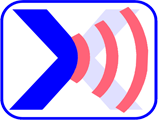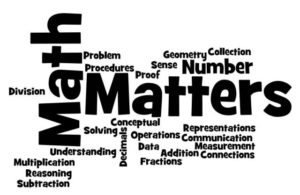Wordy Math Problems1. Brett is twenty-five less than three times the age of Kylie. Kylie is twelve less than two times the age of James. the sum of all of their ages is eighty.How old is James?How old is Kylie?How old is Brett?2. Forty-three times a number plus the sum of seven and the same number is greater than thirteen thousand three hundred forty-one and less than thirteen thousand four hundred ninety-three.  How many integers satisfy this condition?What integers satisfy this condition (separate integers with commas)?3. Alexander paid \$192.55 for a cell phone after a seventeen percent discount was applied to the price.  What was the original price? (round to the nearest dollar)4.The difference between three hundred sixty-seven and half of a number is three hundred twenty-eight.  What is the number?5. The sum of two numbers is one hundred sixty-six. Three times the smaller number plus eighteen is the larger number.  What are the two numbers? (smaller number, larger number)6. Jonathan has a total of one hundred forty-seven nickels, dimes and quarters. He has a total of \$17.45. He has fifteen more nickels than quarters and twelve more dimes than nickels.  How much of each coin does he have? (Nickels, Dimes, Quarters)7. William has a 50% silver alloy. Anna has a 55% silver alloy. They melted their alloy together to create one hundred fifty-five grams of 52% silver alloy.  How many grams was William’s silver alloy?8. Jessica needs a mixture of 8% oats and 92% corn. she currently has sixteen grams of a mixture containing 75% oats and 25% corn.  How many pounds of corn should be added to the mixture?9. If Megan were two years younger she would be three times the age Deanna. if Megan were eight years older, she would be four times the age of Deanna.  How old is Deanna?10. Jessica sold 21 CDs at \$11.50 each. For each CD sold she made an average profit of one hundred forty-seven cents.  How many more CDs must Jessica sell at \$18.50 each to earn an overall average profit of at least \$4.13 per CD?